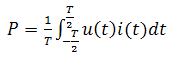`>`首页 > 电工技术 > 电工公式`>`最全的功率计算公式，电工学功率公式大全 正文

# 最全的功率计算公式，电工学功率公式大全

## 1、普遍适用的功率计算公式

p(t)=u(t)i(t)   （1）

p(t)=F(t)v(t)  （2）

P=W/T          （3）
W为时间T内做的功。（4）

S=UI

P=UI
P=U2/R
P=I2R

Q=UIsinφ

P=UIcosφ

P2+Q2=S2

Q=0，S=P

`相关文章`